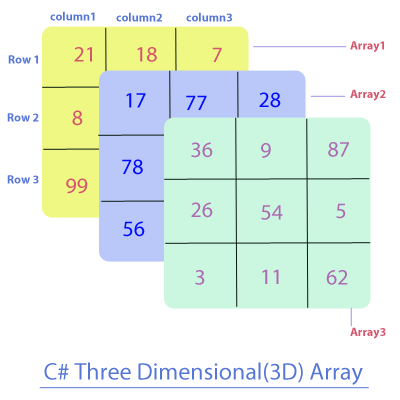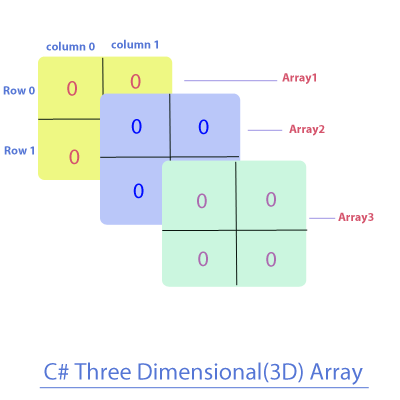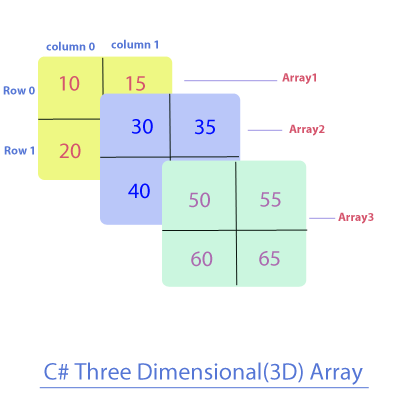Next >

# C# three-dimensional Array

In C# language, a three-dimensional(3D) array is used to contain multiple 2D arrays, where each 2D array is holding values of a same type. All 2D arrays contained in a 3D array can be thought of as stacked one behind another.

In a 3D array, the elements of each 2D array in are arranged in rows and columns, where each element is accessed by using three index values i.e.
• The first index value represents a specific 2D array within our 3D array.
• The second index value represents a row of the 2D array being referred by the first index.
• The third index value represents a column of the 2D array being referred by the first index.
• And all three indexes are separated by a comma i.e. there will be two commas separating 3 indexes, which are representing 3 dimensions, as shown below.## Note:

• A three-dimensional array contains arrays that are holding either of the following values:
• Primitive values of the same type, or
• Object references of the same type.
• An array of any dimension is an object stored on the Heap memory.

We will understand a three-dimensional(3D)array in three separate parts -
• Declaring a 3D array.
• Constructing a 3D array.
• Initializing a 3D array.

## Declaration of a three-dimensional array

The general form of only declaring a three-dimensional array is :

``type[,,] array-name;``
• type is a type of elements this 3D array will hold, followed by a pair of square brackets with double commas in it [ , , ]. These commas are signifying that we are declaring a 3D array and these commas are separating the three dimensions of the it.
• array-name, is the name of the array.

Let us show you an example of declaring a three-dimensional array:
``````//C# Example of a three-dimensional array

using System;

class A
{
public static void Main()
{
//Declaration of a 3D array, which will hold arrays of int values.
int[,,] threeDA1;

//Declaring a 3D array holding arrays of object references of the type class A.
A[,,] threeDA2;
}
}``````

Note: So far, 3D arrays are only declared, hence neither of these 3D arrays(threeA1 and threeDA2) exist on the heap yet and they don't contain any values as of now.

## Construction of a three-dimensional array

The general form of declaring and constructing a three-dimensional array is :

``type[,,] array-name = new type[first-dimension , second-dimension, third-dimension]``
where:
• type is a type of elements this array will hold, followed by a pair of square brackets with two commans in it [,,]. These two commas are signifying that we are declaring a 3D array.
• array-name, is the name of the array.
• new is the keyword using which an array is constructed and gets the memory allocation.
• first-dimension represents the total number of 2D arrays contained in this 2D array.
• second-dimension represents the total number of rows in each 2D array in a 3D array.
• third-dimension represents the total number of columns in each 2D array in a 3D array.

Note:The total number of elements in a 3D array can be calculated by multiplying all the three dimensions.

An Example of constructing a three-dimensional array.
``````//C# Example of constructing a three-dimensional array

using System;

class A
{
public static void Main()
{
//A 3D Array is constructed on the heap with a size to hold 3 2D int arrays.
//Where each array has 2 rows and 2 columns in it.
int[,,] twoDA1= new int[3,2,2];
}
}``````

Note: If a 3D array that contains 2D arrays with numeric primitive values, is not initialized with values then each of its 2D arrays is automatically initialized with zero values. The total number of elements in a 3D array can be calculated by multiplying all the three dimensions, therefore, the total number of elements in our example will be 3x2x2 = 12 elements.

Post the construction of our 3D array, its 2D arrays and their elements are depicted in the diagram below.Our 3D int array is only constructed and we have not manually initialized its elements yet, therefore, the elements of its 2D arrays are initialized with their default values:
• An array holding any numeric primitive type values will be automatically initialized to zero(0), and,
• An array holding object references will be automatically initialized to null.
Please look at the table to know more -

Array type Default value
char '\u0000'
byte 0
short 0
int 0
long 0
float 0
double 0
bool false
String null
Object null

## Initialization of a two-dimensional array

Our 3D int array created in the last example contains 3 automatically initialized 2D arrays, so let's manually initialize each of the 3 2D arrays with int values. To do this we will have to access all the three dimensions of a 3D array.

• First we will access each of the three 2D int arrays by using their index position(starting with index 0), which is specified by the first-dimension, where:
• The reference to the first array is specified by its index i.e. 0.
• The reference to the second array is specified by its index i.e. 1.
• The reference to the third array is specified by its index i.e. 2.
• Next, we will use the second-dimension which represents a row(starting with index 0) of a 2D array(represented by the first-dimension)
• And finally, we will use the third-dimension which represents a column(starting with index 0) of a 2D array(represented by the first-dimension).

``````//C# Example of initialization of a three-dimensional array

using System;

class A
{
public static void Main()
{
//A 3D array holding three 2D int arrays(each array holding 2 rows and 2 columns of int values).
int[,,] threeDA= new int[3,2,2];

threeDA[0,0,0]=10; //inserting 10 in the first(0th index) array at its 0th row and 0th column index
threeDA[0,0,1]=15; //inserting 15 in the first(0th index) array at its 0th row and 1st column index
threeDA[0,1,0]=20; //inserting 20 in the first(0th index) array at its 1st row and 0th column index
threeDA[0,1,1]=25; //inserting 25 in the first(0th index) array at its 1st row and 1st column index

threeDA[1,0,0]=30; //inserting 30 in the second(1st index) array at its 0th row and 0th column index
threeDA[1,0,1]=35; //inserting 35 in the second(1st index) array at its 0th row and 1st column index
threeDA[1,1,0]=40; //inserting 40 in the second(1st index) array at its 1st row and 0th column index
threeDA[1,1,1]=45; //inserting 45 in the second1stindex) array at its 1st row and 1st column index

threeDA[2,0,0]=50; //inserting 50 in the third(2nd index) array at its 0th row and 0th column index
threeDA[2,0,1]=55; //inserting 55 in the third(2nd index) array at its 0th row and 1st column index
threeDA[2,1,0]=60; //inserting 60 in the third(2nd index) array at its 1st row and 0th column index
threeDA[2,1,1]=65; //inserting 65 in the third(2nd index) array at its 1st row and 1st column index

}
}``````

Depicting the effect of the code above, which has initialized a 3D array.Now let's see how a 2D array within 3D array is initialized with values -

• ``twoDA[0,0,0]=10;``
The index position of the first 2D array in our 3D array is specified by the first-dimension i.e. zero, followed by a comma, followed by the index position the row in the first array specified by the second-dimension i.e. zero, followed by a comma, followed by the index position the column in the first array specified by the third-dimension i.e. zero, at which we are inserting an int value 10.

• ``threeDA[0,0,1]=15``
We are inserting 15 in the first(0th index) array at its 0th row and 1st column index.

• ``threeDA[0,1,0]=20``
We are inserting 20 in the first(0th index) array at its 1st row and 0th column index .

• ``threeDA[0,1,1]=25``
We are inserting 20 in the first(0th index) array at its 1st row and 1st column index . And like this, we have inserted the elements in the next two 2D arrays as well.

## Reading a 2-D array holding arrays of primitive values.

We can read any element of an array contained in a 3D array using its index position in the array. For example, to read and display an element at the 0th row and 0th column of 1st 2D array in our 3D array, it is simply -

``Console.WriteLine(twoDA[0,0,0]);	//Reading the first(1st index) array and its zeroth(0th index) element``

We read the elements of a 1D array by using a single for-loop, and we can we read all the elements of a 2D array by using 2 nested for-loops, and to read all the elements of a 3D array, we will use 3 nested for-loops in the following way:
• Outer for-loop tracks through all the 2D arrays contained in a 3D array, starting with a 2D array at index 0.
• The next nested for-loop tracks through all the rows in the current 2D array.
• The last nested for-loop tracks through all the columns in the current 2D array.

``````//C# Example of reading a 3D array holding 2D arrays of primitive values.

using System;

class A
{
public static void Main()
{
//A 3D array holding three 2D int arrays(each array holding 2 rows and 2 columns of int values).
int[,,] threeDA= new int[3,2,2];

threeDA[0,0,0]=10; //inserting 10 in the first(0th index) array at its 0th row and 0th column index
threeDA[0,0,1]=15; //inserting 15 in the first(0th index) array at its 0th row and 1st column index
threeDA[0,1,0]=20; //inserting 20 in the first(0th index) array at its 1st row and 0th column index
threeDA[0,1,1]=25; //inserting 25 in the first(0th index) array at its 1st row and 1st column index

threeDA[1,0,0]=30; //inserting 30 in the second(1st index) array at its 0th row and 0th column index
threeDA[1,0,1]=35; //inserting 35 in the second(1st index) array at its 0th row and 1st column index
threeDA[1,1,0]=40; //inserting 40 in the second(1st index) array at its 1st row and 0th column index
threeDA[1,1,1]=45; //inserting 45 in the second1stindex) array at its 1st row and 1st column index

threeDA[2,0,0]=50; //inserting 50 in the third(2nd index) array at its 0th row and 0th column index
threeDA[2,0,1]=55; //inserting 55 in the third(2nd index) array at its 0th row and 1st column index
threeDA[2,1,0]=60; //inserting 60 in the third(2nd index) array at its 1st row and 0th column index
threeDA[2,1,1]=65; //inserting 65 in the third(2nd index) array at its 1st row and 1st column index

//Checking the total numbers of elements in our 3D array
//By using the Length property of array
Console.WriteLine("Length of our 3D array is : " + threeDA.Length);
Console.WriteLine("Contents of this array are : ");

//Reading our 3D array by using nested for-loops
for(int array=0; array<3; array++)
{
for(int row=0; row<2; row++)
{
for(int column=0; column<2; column++)
{
Console.WriteLine(threeDA[array,row, column]);
}
}
}
}

}```
```

## Output-

``````Length of our 3D array is : 12
Contents of this array are :
10
15
20
25
30
35
40
45
50
55
60
65```
```

## 2-D Array holding arrays of object references

A three-dimensional array may also hold 2D arrays that are holding object references of the same type. Let's see a coding example -

``````//C# Example of reading a 3D array holding 2D arrays of object references

using System;

class A
{
public static void Main()
{
//A 3D array holding three 2D arrays(each array holding 2 rows and 2 columns of object references of class A).
A[,,] threeDA= new A[3,2,2];

threeDA[0,0,0] = new A(); //inserting an object of A in the first(0th index) array at its 0th row and 0th column index
threeDA[0,0,1] = new A(); //inserting an object of A in the first(0th index) array at its 0th row and 1st column index
threeDA[0,1,0] = new A(); //inserting an object of A in the first(0th index) array at its 1st row and 0th column index
threeDA[0,1,1] = new A(); //inserting an object of A in the first(0th index) array at its 1st row and 1st column index

threeDA[1,0,0] = new A(); //inserting an object of A in the second(1st index) array at its 0th row and 0th column index
threeDA[1,0,1] = new A(); //inserting an object of A in the second(1st index) array at its 0th row and 1st column index
threeDA[1,1,0] = new A(); //inserting an object of A in the second(1st index) array at its 1st row and 0th column index
threeDA[1,1,1] = new A(); //inserting an object of A in the second1stindex) array at its 1st row and 1st column index

threeDA[2,0,0] = new A(); //inserting an object of A in the third(2nd index) array at its 0th row and 0th column index
threeDA[2,0,1] = new A(); //inserting an object of A in the third(2nd index) array at its 0th row and 1st column index
threeDA[2,1,0] = new A(); //inserting an object of A in the third(2nd index) array at its 1st row and 0th column index
threeDA[2,1,1] = new A(); //inserting an object of A in the third(2nd index) array at its 1st row and 1st column index

//Checking the total numbers of elements in our 3D array
//By using the Length property of array
Console.WriteLine("Length of our 3D array is : " + threeDA.Length);
Console.WriteLine("Contents of this array are : ");

//Reading our 3D array by using nested for-loops
for(int array=0; array<3; array++)
{
for(int row=0; row&;t;2; row++)
{
for(int column=0; column<2; column++)
{
Console.WriteLine(threeDA[array,row, column]);
}
}
}
}

}``````

## Output-

``````Length of our 3D array is : 12
Contents of this array are :
A
A
A
A
A
A
A
A
A
A
A
A``````

## Program Analysis

Over here, we have created a 3D array to hold 3 2D arrays, with each array is holding 4 references to objects of type A.

## A 3D array holding object references will be automatically initialized to null

A 3D array holding object references if left uninitialized will be automatically initialized to null.

``````//C# A 3D array holding object references will be automatically initialized to null

using System;

class A
{
public static void Main()
{
//A 3D array holding three 2D arrays(each array holding 2 rows and 2 columns of object referenced of class A).
A[,,] threeDA= new A[3,2,2];

//Checking the total numbers of elements in our 3D array
//By using the Length property of array
Console.WriteLine("Length of our 3D array is : " + threeDA.Length);
Console.WriteLine("Contents of this array are : ");

//Reading our 3D array by using nested for-loops
for(int array=0; array<3 array++)
{
for(int row=0; row<2; row++)
{
for(int column=0; column<2; column++)
{
if( threeDA[array,row,column]== null)
Console.WriteLine(threeDA[array,row, column] + "A null element");
}
}
}
}

}``````

## Output:

``````Length of our 3D array is : 12
Contents of this array are :
A null element
A null element
A null element
A null element
A null element
A null element
A null element
A null element
A null element
A null element
A null element
A null element``````

As you can see in the output of our last example, a 3D array to hold objects of type A is constructed on the heap and its elements are not manually initialized by us, therefore, the elements of this array will be automatically initialized to null, which means that each reference in this 3D array points to no value i.e. because object was not initialized, and therefore, nothing is printed when the null value is printed.